5.5.1. Isothermal distributions

The simplest distribution of gas temperatures would be an isothermal distribution, with Tg being constant. The intracluster gas would become isothermal if thermal conduction were sufficiently rapid (see equation 5.41 for the relevant time scale). Alternatively, the gas may have been introduced into the cluster with an approximately constant temperature, and its thermal distribution be unchanged since that time. Lea et al. (1973) fit the gas distributions in the Coma, Perseus, and M87/Virgo clusters, assuming that the gas distribution were self-gravitating and isothermal. If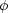(r) is due only tog, then equation (5.56) and Poisson's equation for the gravitational potential are equivalent to equation (2.9), the equation of an isothermal sphere. Note, however, that this is not trivially true, since equation (2.9) was derived from the stellar dynamical equation for a collisionless gas, while equation (5.56) is the hydrostatic equation for a collisionally dominated fluid. Lea et al. therefore used equation (5.57) to fit the gas densityg. This is not consistent, since the gas masses derived from these fits were generally less than 20% of the virial mass of the cluster, and the core radii derived were larger than those of the galaxies (Section 4.4).

More consistent isothermal models can be derived if the gravitational potential of the cluster is not assumed to come only from the gas (Cavaliere and Fusco-Femiano, 1976, 1978; Sarazin and Bahcall, 1977). Equation (5.56) can be written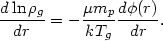(5.62)

If the potential is given by equations (5.59) and (5.61), then the gas distribution is given by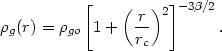(5.63)

Here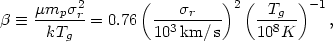(5.64)

where the numerical value follows if solar abundances are assumed so that µ = 0.63. Another way to derive equation (5.63) is to note that if the galaxy velocity dispersion is isotropic, the galaxy densitygal in a cluster will be given by equation (5.62), replacing kTg / µmp withr2. Then eliminating the potential between the equations forg andgal yieldsggal, and equation (5.63) follows if the galaxy distribution is given by equation (5.57) (Cavaliere and Fusco-Femiano, 1976).

This self-consistent isothermal model (equation 5.63) assumes that the gas and galaxy distributions are both static and isothermal and that the galaxy and total mass distributions are identical. While none of these assumptions is fully justified, and the gas is probably not generally isothermal, this model has the advantage that the resulting gas distribution is analytic and that basically all the integrals needed to compare the model to the observations of clusters are also analytic. For example, the total gas mass and emission integral (equation 5.20) are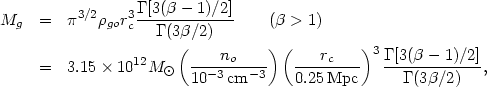(5.65)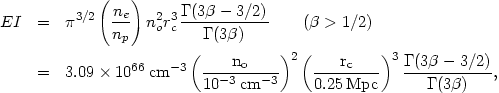(5.66)

where n0 is the central proton density,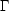is the gamma function, and solar abundances have been assumed in deriving the numerical values. The values ofin the parentheses give the limits such that the appropriate integrals converge at large radii. Similarly, the X-ray surface brightness at a projected radius b is proportional to the emission measure EM, defined as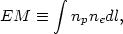(5.67)

where l is the distance along the line-of-sight through the cluster at a projected radius b. Then, for the self-consistent isothermal model, the emission measure is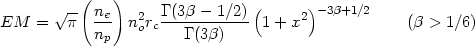(5.68)

where xb/rc. The microwave diminution (Section 3.5) at low frequencies is given by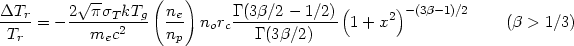(5.69)

whereT is the Thomson cross section, and Tr is the cosmic background radiation temperature. Numerically,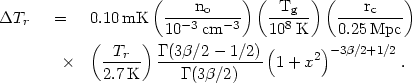(5.70)

Equation (5.68) has been used extensively to model the surface brightness I(b) of the X-ray emission from clusters (Gorenstein et al., 1978; Branduardi-Raymont et al., 1981; Abramopoulos and Ku, 1983; Jones and Forman, 1984; Section 4.4.1). The most accurate data have come from the Einstein X-ray satellite, which was sensitive only to low energy X-rays (h< 4 keV). For high temperature gas (Tg3 × 107 K), the low energy X-ray emissivity is nearly independent of temperature, and thus I(b)EM even if the gas temperature varies. Large surveys of X-ray distributions fit by equation (5.68) have been made by Abramopoulos and Ku (1983), who set= 1 (equal gas and galaxy distributions), and Jones and Forman (1984), who allowedto vary. Figure 16 shows the data on the X-ray surface brightness of three clusters from Jones and Forman (1984) and their best fit models using equation (5.68). Equation (5.68) is a good fit to the majority of clusters, but fails in the central regions of some clusters, possibly because these clusters contain cooling accretion flows (Sections 4.3.3, 4.4.1, and 5.7). The average value ofdetermined by fits to the X-ray surface brightness of a large number of clusters was found to be (Jones and Forman, 1984)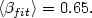(5.71)

Thus the X-ray surface brightness and implied gas density vary on average as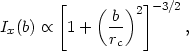(5.72)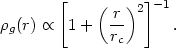(5.73)

This indicates that the gas density should fall off less rapidly with radius than the galaxy density (in agreement with many other observations, such as Abramopoulos and Ku, 1983), and that the energy per unit mass is higher in the gas than in the galaxies (Jones and Forman, 1984). For this average value of, the total X-ray luminosity converges, but the total gas mass given by equation (5.65) does not.

Unfortunately, this does not agree with the determinations of the X-ray spectral temperatures and the galaxy velocity dispersions of clusters (Mushotzky, 1984). For example, the observed correlation betweenr and Tg in equation (4.10) implies that the average valuedetermined by gas temperatures and galaxy velocity dispersions is <spect>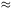1.3.

From a sample of clusters with well-determined spectra, Mushotzky (1984) finds <spect>1.2, which he notes is about twice the value determined from observations of the X-ray surface brightness. While Jones and Forman (1984) argue that their values offit are in excellent agreement with the determinations from spectral observations, in fact their data show that the two values do not agree to within the errors in the majority of cases. For the clusters they studied <spect>1.1. Thus the general result seems to be that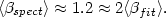(5.74)

A number of suggestions have been made as to the origin of this discrepancy. First, the gas may very well not be isothermal. However, Mushotzky (1984) has argued that the same problem occurs for other thermal distributions in the gas. Second, it may be that the line-of-sight velocity dispersion does not represent accurately the energy per unit mass of the galaxies. Equation (5.63) assumes that the galaxy velocity distribution is isotropic. The distribution could be anisotropic, either if the cluster is highly flattened (Section 2.9.3) or if the cluster is spherical but galaxy orbits are largely radial (rather than having a uniform distribution of eccentricities; see Section 2.8). A detailed study (Kent and Sargent, 1983) of the positions and velocities of galaxies in the Perseus cluster has produced a more accurate description of the cluster potential and significantly reduced the discrepancy, although it still is significant. Third, it may be that many of the galaxy velocity dispersions measured for clusters are contaminated by foreground or background groups (Geller and Beers, 1982). The velocity dispersions may also be affected by subclustering or nonvirialization of the cluster. All of these effects will cause the data to overestimate the actual velocity dispersion (the cluster potential), and thus to overestimatespect. Perhaps one indication that such systematic errors in the velocity dispersion might be occurring is that Coma, the best studied regular cluster, does not show adiscrepancy. Another possible solution to thediscrepancy would be if the gas dominated the total cluster mass at large radii (Henriksen and Mushotzky, 1985; Section 5.5.5); this would invalidate the assumption that the galaxies and gas were test particle distributions in the missing mass potential.

Finally, let me point out an utterly trivial possible explanation of thediscrepancy. The particular form of the gas distribution in the self-consistent isothermal model as given above (equations 5.63 through 5.70) depends on assuming that the cluster potential is fit by the King approximation to the isothermal sphere (equations 5.59 and 5.61). This approximation breaks down at large radii, where the King model density varies asr-3 while a real isothermal sphere density varies asr-2. Of course, it is the gas distribution at large radii which has the greatest leverage in affecting the fit to equation 5.63. If the mass density in clusters is really isothermal, then equation 5.63 will not fit the observed gas distribution for the correct value of. As an alternative, let us assume that the cluster mass distribution can be fit by the simple analytic form(r) =0(1 + x2)-1, which is similar to equation (5.57) but has the correct asymptotic form for an isothermal sphere. Then all the formulae for the self-consistent isothermal sphere remain unchanged if we substitute(2/3)in equations (5.63) through (5.70). Retaining the present definition of, this is equivalent tofit = (2/3)spect, which is essentially consistent with the observations. The observed <fit>2/3 implies <spect>1 which is consistent with the spectral observations. Another way of expressing all this is to say that the observed gas distribution (equation 5.73) is essentially that of an isothermal sphere.

This possible discrepancy between the globally determined X-ray temperatures of clusters and their surface brightness will probably only be resolved when spatially resolved X-ray spectra are available, allowing a simultaneous determination of the spatial variation of the gas density and temperature (Chapter 6).

One of the derivations of the self-consistent isothermal model involves noting that the galaxy distribution also solves the hydrostatic equation if the galaxy velocity dispersion is isotropic (see discussion following equation (5.64)). If the galaxy distribution is spherical, but the galaxy velocity dispersions in the directions parallel to the cluster radius (r) and transverse to that direction (t) are different, this derivation fails. However, for a constant (isothermal) galaxy velocity dispersion and a constant anisotropy of the velocity dispersion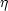1 -t2 /r2, the gas density is given byg(gal r2)(White, 1985).

Finally, Henry and Tucker (1979) have pointed out the existence of a simple relationship (Lx rc)2/5Tg between X-ray temperatures, luminosities, and core radii in clusters based on the isothermal model.